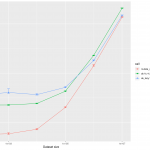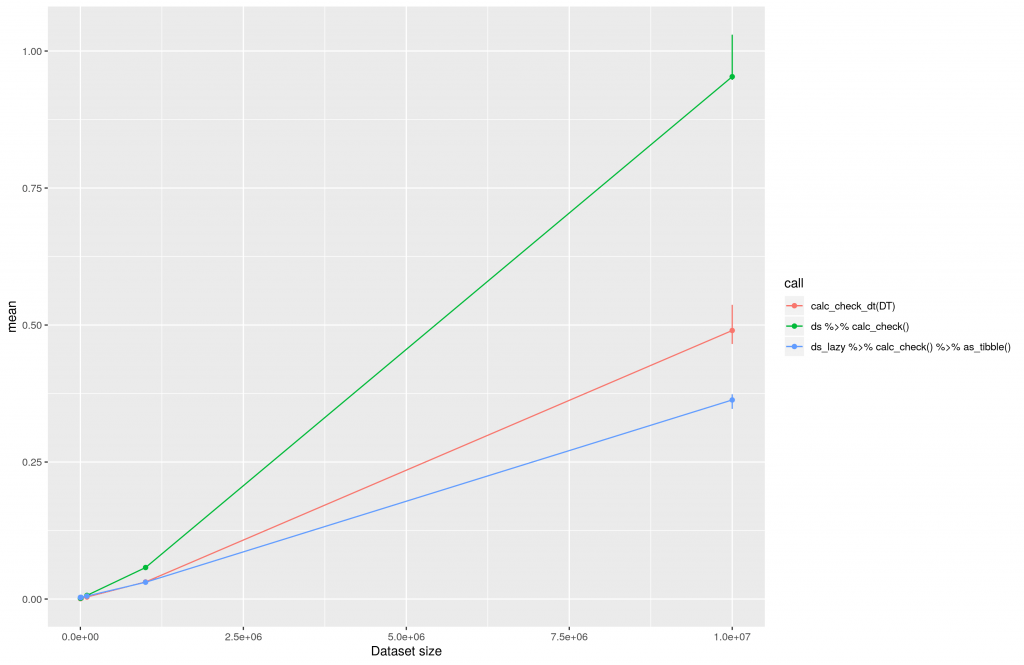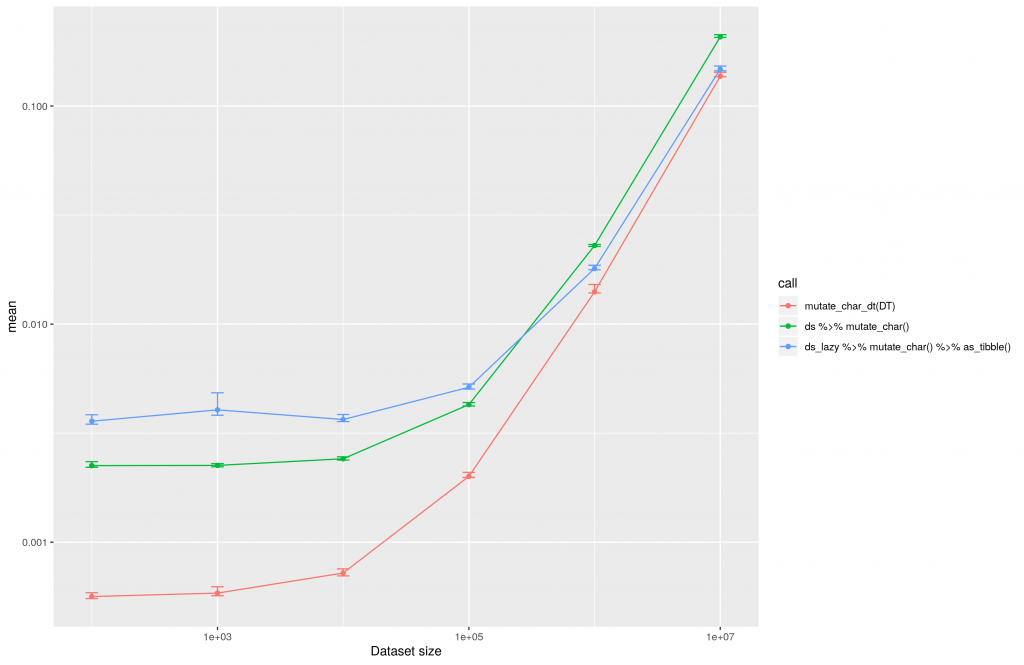Want to share your content on R-bloggers? click here if you have a blog, or here if you don't.### When Hadley Wickham published dtplyr 1.0.0 last week, I was super excited. Moreover, I wanted to know, if it really is a useful package. Therefore I performed some tiny research being presented in this blog entry.

This article was updated at December 2nd 2019 due to upcoming issues: https://github.com/zappingseb/dtplyrtest/issues?q=is%3Aissue+is%3Aclosed
Many thanks to:
Stefan Fritsch, Dirk Raetzel, Jan Gorecki for qualified review of the article and the code.

Ok. So dtplyr gives you the speed of data.table with the nice API of dplyr. This is the main feature. You will see this in the following code. The speedup of dtplyr should allow converting variables in large scale data sets by simple calls. So I first constructed an example call which is partly taken from Hadley Wickhams blog entry:

n <- 100
# Create a word vector
words <- vapply(1:15, FUN = function(x) {
paste(sample(LETTERS, 15, TRUE), collapse = "")
}, FUN.VALUE = character(1))

# Create an example dataset
df <- data.frame(
word = sample(words, n, TRUE),
letter = sample(LETTERS, n, TRUE),
id = 1:n,
numeric_int = sample(1:100, n, TRUE),
numeric_double = sample(seq(from = 0, to = 2, by = 1/(10^6)), n, TRUE),
missing = sample(c(NA, 1:100), n, TRUE)
)

# Convert the numeric values
df %>%
filter(!is.na(missing)) %>%
group_by(letter) %>%
summarise(n = n(), delay = mean(numeric_int))


The call shown is the one used within dplyr. In dtplyr a lazy evaluation term needs to be added. At the end of the dplyr call a %>% as_tibble() is needed to start the evaluation. In data.table the call looks totally different.

# dtplyr call
ds_lazy <- lazy_dt(ds)
ds_lazy %>% fil.... %>%
as_tibble()

DT <- as.data.table(ds)
# data.table call
DT_int[!is.na(missing), .(n = .N, delay = mean(numeric_int)), letter]


To evaluate the execution I created testing datasets of different sizes. The smallest dataset will contain 100 rows. The largest dataset 10E7 rows. Afterward I ran the data conversion operations and logged the time of execution using the bench package and 1000 iterations. All calculations were performed on an AWS t3.2xlarge machine hosting the rocker/tidyverse docker image. This is the result of the calculations plotted in a linear manner:The execution time of data transformations using dplyr, dtplyr and data.table plotted in a linear manner.

One can clearly see that dtplyr (ds_lazy …) and data.table (red line, calc_check_dt) increase nearly linear over time. Both seem to be really good solutions when it comes to large datasets. The difference of both at 10E7 can be explained by the low number of iterations being used at benchmarking. This post should not provide perfect benchmarks, but rather some fast insights into dtplyr. Thus, a reasonable computing time was preferred.

Please consider, conversion and copying of a large data.frame into a data.table or tibble may take some time. This shall be considered. When using data.table all datasets should be data.tables right from the start.

From the linear plot you cannot see at what point it is useful to use dtplyr against dplyr. This can thus be seen in the following plot:The execution time of dtplyr, dplyr and data.table plotted for the conversion of different datasets in a logarithmic manner.

The above image clearly shows that it is worth using dtplyr over dplyr in case there are more than 10E4 rows inside the dataset. So if you never work with datasets of this size it might not be worth considering dtplyr at all. On the other hand, you can see the data.table package itself is always the fastest solution.

As in my project we do not just deal with numeric transformations of data, but also character transformations, I wanted to know if the dtplyr package could be helpful for this. The conversion I performed for character strings looks like this:

# dplyr code
df %>%
filter(letter == "A") %>%
mutate(word_new = paste0(word, numeric_int)) %>%
select(word_new)

# data.table code
DT[letter == "A", .(word_new = paste0(word, numeric_int))]
`

The calculation results are similar to those gained with numeric conversions

Inside this plot the difference between dplyr and data.table is nearly invisible. All methods increase the computing time in linear relation to the data set size.The execution time of large scale data conversions for character evaluations with dtplyr, dplyr and data.table.

For character transformations the performance of dplyr is pretty good. It is just useful to use dtplyr for datasets with >10E5 rows. In case of the largest dataset tested in this approach with 10E7 rows, dtplyr is twice as fast as dplyr.

### Conclusion

The code you need to write to use dtplyr is really simple. Especially if you are like me dplyr native and do not want to start using data.table. But there will be a lot of use-cases where it is not necessary or not even useful to use the dtplyr package. So before you start writing fancy dtplyr pipelines, think about the necessity.

All code for this project is available at: https://github.com/zappingseb/dtplyrtest – Please see updates from last commit